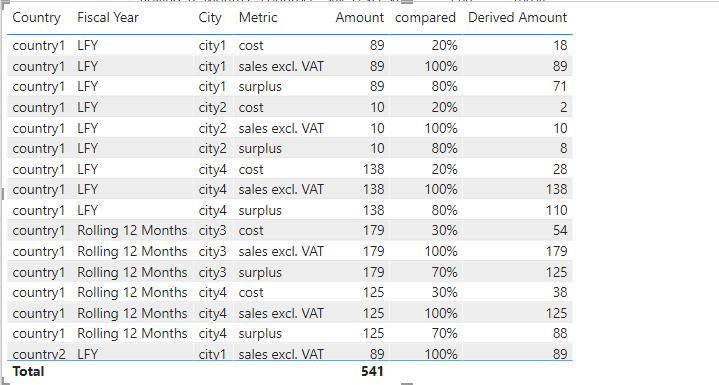cancel
Showing results for
Did you mean:

Fabric is Generally Available. Browse Fabric Presentations. Work towards your Fabric certification with the Cloud Skills Challenge.## Calculate percentages that will later be used to derive sales amounts in another table?

Hello,

Here is the sample data:

For the same data, I have 2 questions (highlighted below in red) that I've been struggling with for a long time. I'd greatly appreciate your help.

Background:

Question 1: I created the following calculated table (Calculated Table A) from dataset X, which summarizes sales amount by Fiscal Year, Country, Metric. How do I create the "% compared to Sales excl. VAT" column as highlighted in red?

"% compared to Sales excl. VAT" is defined as: For each Country and Fiscal Year, all Metrics are divided by "sales excl. VAT". For example, the 2nd row's expected value will be: 20/100 = 20%.

Calculated Table A (from dataset X, COUNTRY granularity)

 Fiscal Year Country Metric Amount % compared to Sales excl. VAT LFY country1 sales excl. VAT 100 100% LFY country1 cost 20 20% LFY country1 surplus 80 80% Rolling 12 Months country1 sales excl. VAT 200 100% Rolling 12 Months country1 cost 60 30% Rolling 12 Months country1 surplus 140 70% LFY country2 sales excl. VAT 150 100% LFY country2 surplus 90 60% Rolling 12 Months country2 sales excl. VAT 180 100% Rolling 12 Months country2 surplus 80 44%

Question 2: I also created Calculated Table B (from a different dataset Y), which also summarizes sales amount by Fiscal Year, Country, Metric, but on City level. Another difference compared to the first table is that, Metric column in this table has only 1 value: "Sales excl. VAT".

Calculated Table B (from dataset Y, CITY granularity)

 Fiscal Year Country City Metric Amount LFY country1 city1 sales excl. VAT 89 LFY country1 city2 sales excl. VAT 10 Rolling 12 Months country1 city3 sales excl. VAT 179 LFY country2 city4 sales excl. VAT 138 Rolling 12 Months country2 city4 sales excl. VAT 125

Now, using Calculated Table B as the base, how do I add Derived Amount column (below in red)?

Basically, to calculate Derived Amount, I need to:

• first bring in all Metric values that appear in Calculated Table A (meaning not just "Sales excl. VAT", but also "Cost" and "Surplus"), as seen in the Metric column below. I guess this would require CROSSJOIN?;
• then bring the corresponding % values in the 1st question into the "% compared to Sales excl. VAT" column, as seen below. The assumption is that Cities share the same percentages as their corresponding Countries;
• then Derived Amount = [Amount of Sales excl. VAT] * [% compared to Sales excl. VAT]

Example expected output:

 Country Fiscal Year city Metric Amount % compared to sales excl. VAT Derived Amount country1 LFY city1 sales excl. VAT 89 100% 89 country1 LFY city1 cost null 20% 18 country1 LFY city1 surplus null 80% 71 country2 LFY city4 sales excl. VAT 138 100% 138 country2 LFY city4 surplus null 60% 83 …

Thanks!

1 ACCEPTED SOLUTIONCommunity Support

Hi @haminguyen ,

First, Establishing the relationship between the two tablesSecond, create a column.

``Column = IF('Table A'[Metric]in {"cost","surplus"},'Table A'[Amount],BLANK())``

Last, create measures.

``````compared = var sum_a=CALCULATE(SUM('Table A'[Column]),FILTER(ALL('Table A'),'Table A'[Country]=SELECTEDVALUE('Table A'[Country])&&'Table A'[Fiscal Year]=SELECTEDVALUE('Table A'[Fiscal Year])))
var big= IF(SELECTEDVALUE('Table A'[Metric])in {"sales excl. VAT"},SELECTEDVALUE('Table A'[Amount]),BLANK())
VAR BIG_COMPARE = CALCULATE(MAX('Table A'[Amount]),FILTER(ALL('Table A'),'Table A'[Country]=SELECTEDVALUE('Table A'[Country])&&'Table A'[Fiscal Year]=SELECTEDVALUE('Table A'[Fiscal Year])))
var result=IF(BIG_COMPARE>sum_a,BIG_COMPARE,sum_a)
var compare_=SELECTEDVALUE('Table A'[Amount])/result
return compare_````````````Derived Amount = IF([compared]=1,SELECTEDVALUE('Table  B'[Amount]),[compared]*SELECTEDVALUE('Table  B'[Amount]))
``````Best Regards

Community Support Team _ Polly

If this post helps, then please consider Accept it as the solution to help the other members find it more quickly.

2 REPLIES 2Community Support

Hi @haminguyen ,

First, Establishing the relationship between the two tablesSecond, create a column.

``Column = IF('Table A'[Metric]in {"cost","surplus"},'Table A'[Amount],BLANK())``

Last, create measures.

``````compared = var sum_a=CALCULATE(SUM('Table A'[Column]),FILTER(ALL('Table A'),'Table A'[Country]=SELECTEDVALUE('Table A'[Country])&&'Table A'[Fiscal Year]=SELECTEDVALUE('Table A'[Fiscal Year])))
var big= IF(SELECTEDVALUE('Table A'[Metric])in {"sales excl. VAT"},SELECTEDVALUE('Table A'[Amount]),BLANK())
VAR BIG_COMPARE = CALCULATE(MAX('Table A'[Amount]),FILTER(ALL('Table A'),'Table A'[Country]=SELECTEDVALUE('Table A'[Country])&&'Table A'[Fiscal Year]=SELECTEDVALUE('Table A'[Fiscal Year])))
var result=IF(BIG_COMPARE>sum_a,BIG_COMPARE,sum_a)
var compare_=SELECTEDVALUE('Table A'[Amount])/result
return compare_````````````Derived Amount = IF([compared]=1,SELECTEDVALUE('Table  B'[Amount]),[compared]*SELECTEDVALUE('Table  B'[Amount]))
``````Best Regards

Community Support Team _ Polly

If this post helps, then please consider Accept it as the solution to help the other members find it more quickly.Hello, does anyone have any idea?Announcements#### Power BI Monthly Update - November 2023

Check out the November 2023 Power BI update to learn about new features.#### Fabric Community News unified experience

Read the latest Fabric Community announcements, including updates on Power BI, Synapse, Data Factory and Data Activator.#### The largest Power BI and Fabric virtual conference

130+ sessions, 130+ speakers, Product managers, MVPs, and experts. All about Power BI and Fabric. Attend online or watch the recordings.Top Solution Authors
Top Kudoed Authors
Users online (3,042)